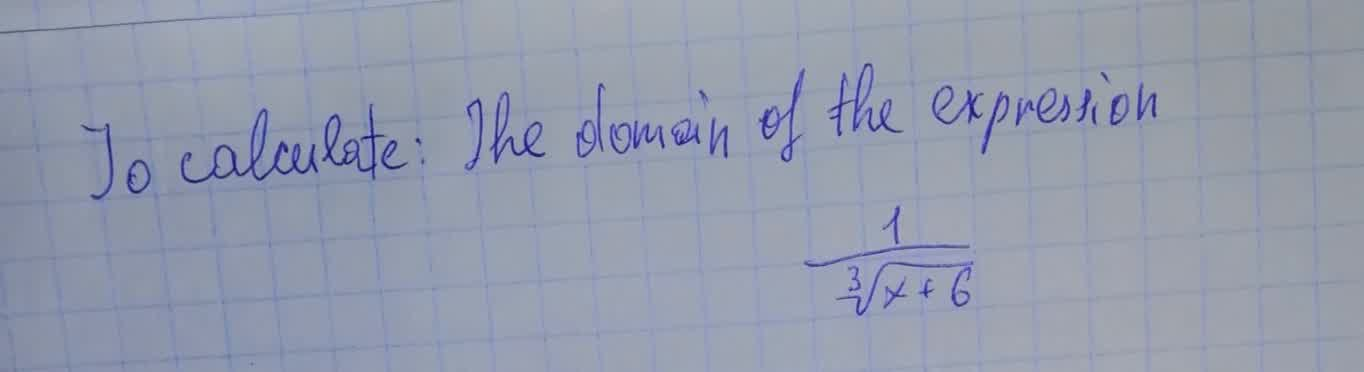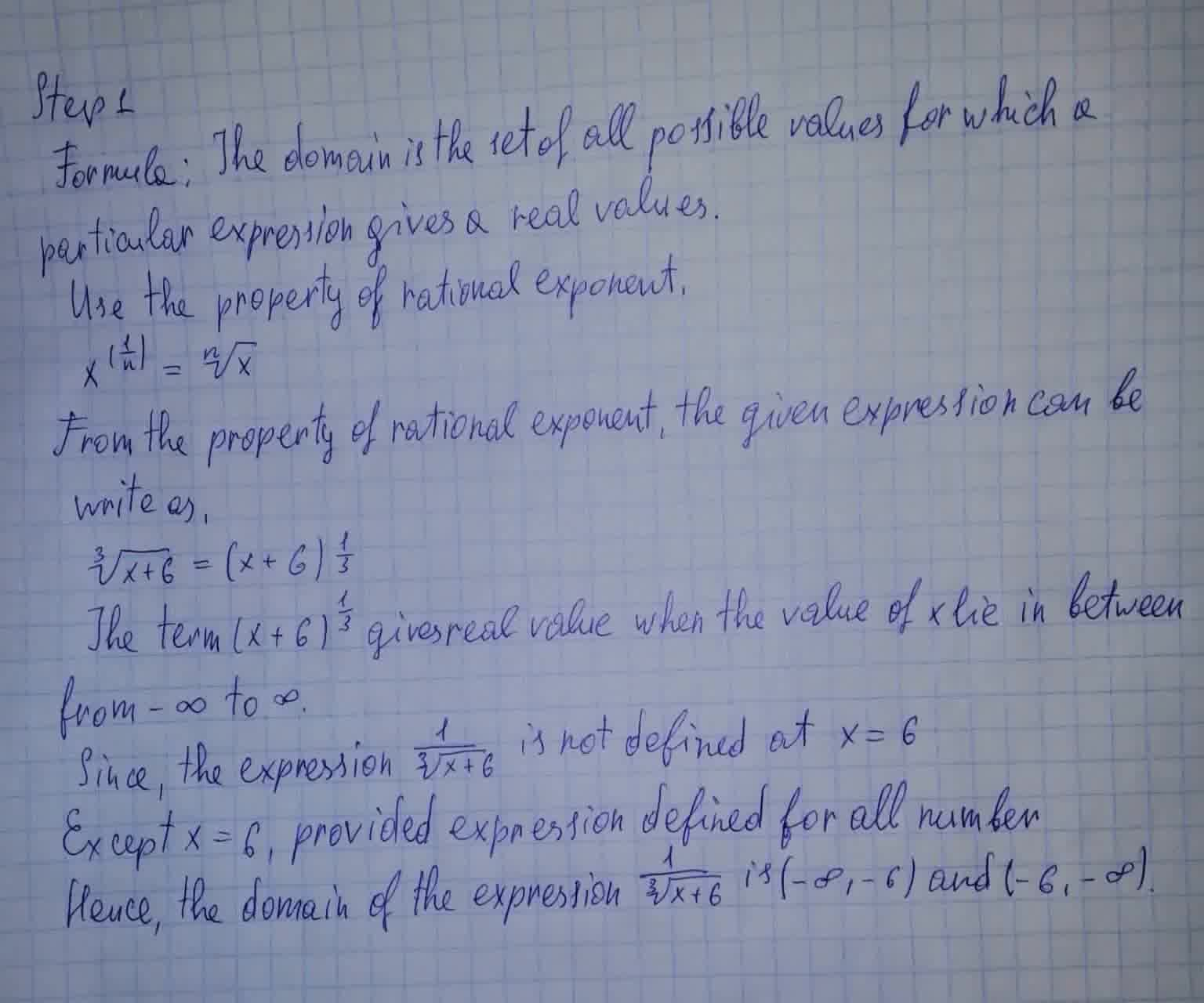# To calculate : The domain of the expression \frac{1}{\sqrt{x+6}}Wierzycaz 2021-08-11 Answered

To calculate : The domain of the expression $$\frac{1}{\sqrt{x+6}}$$• Questions are typically answered in as fast as 30 minutes

### Plainmath recommends

• Get a detailed answer even on the hardest topics.
• Ask an expert for a step-by-step guidance to learn to do it yourself.Laith Petty
Calculation: